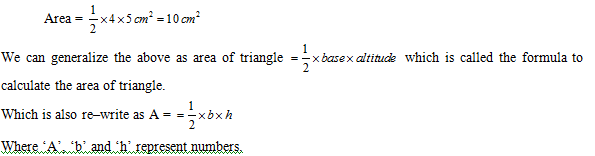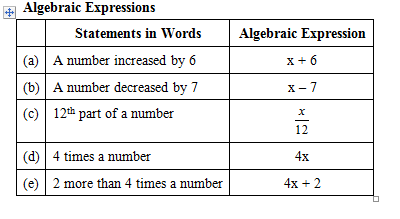# Introduction

## Introduction

We have studied the numbers and learned how to apply the operations like addition, subtraction, etc. on numbers.

For examples, if the base and altitude of a triangle are 4 cm and 5 cm respectively, then the area of the triangle is### Algebra

Now, suppose if at present Sonu’s age is x years and her mother is 5 times of her age, then her mother’s present age should be equal to 5 x years.

Again, Sonu’s age 3 years later = (x+ 3) years

her mother’s age 3 years later = (5x + 3) years

Thus, in arithmetic when we form a particular situation to a general result, we obtain a formula. A formula is written with the help of literals, constants and four basic operations (addition, subtraction, multiplication and division). Using literals in generalized results of arithmetic is nothing but known as algebra.